## STABILITY ANALYSIS OF MAGLEV VEHICLE WITH DELAYED POSITION FEEDBACK CONTROL 1)

Wu Han, Zeng Xiaohui2), Shi Hemu

University of Chinese Academy of Sciences, Beijing 100049, China

 基金资助: 国家自然科学基金.  51805522国家自然科学基金.  11672306国家自然科学基金.  51490673国家重点研发计划课题.  2016YFB1200602中科院先导专项.  XDB22020100中科院信息化专项.  XXH13506-204

Received: 2018-10-9   Accepted: 2018-12-17   Online: 2019-03-18

2)曾晓辉,研究员,主要研究方向：车辆动力学、波流与海洋结构的相互作用.E-mail:zxh@imech.ac.cnAbstract

Because of the inherent instability, EMS maglev train requires the application of active control to achieve a stable suspension. In every suspension control loop, there is inevitable time-delay, which may affect the performance of the system. When the time-delay exceeds a critical value, the maglev train will become unstable. Previous studies have proved the existence of the critical time-delay. The relationship between critical time delay in control loop and vehicle parameters (including vehicle speed, position feedback control gains, guideway parameters, and suspension parameters) is studied in this paper. A dynamic model of a maglev vehicle/guideway coupling system is established. The 10 DOF vehicle includes a carbody and four maglev frames. Each maglev frame contains four electromagnets. The guideway is modelled as a series of continuous simply supported Bernoulli-Euler beams. The vibration equation of the guideway is obtained by a modal superposition method. In order to achieve vehicle/guideway coupling, a conventional electromagnetic force model which is linearized in the neighborhood of stable suspension position is adopted. The fourth-order Runge-Kutta method is used to obtain the dynamic response of the vehicle/guideway coupling system. A proportional derivative (PD) control algorithm is used for the feedback control of the electromagnet current. To facilitate the analysis, this paper assumes that all the time-delay occurs in the control loop, and that only the position feedback control loop exists. In order to analyze the motion property when critical delay occurs, we write a simulation program. Using the program, the dynamic responses of maglev vehicle considering different position feedback control delay are calculated. The delay value which results in motion divergence is defined as critical time-delay. Based on the calculations and analysis, following suggestions to promote the stability and weaken the effect of time-delay in position feedback control loop are provided: Enlarge bending rigidity and damping ratio of the guideway; Reduce position feedback control gain; Enlarge velocity feedback control gain; Enlarge second suspension damping. In addition, in view of that the critical time-delay of a stationary vehicle is always smaller than that of a running vehicle, hence, the critical time-delay of stationary vehicle shall be considered as safety limit value.

Keywords： EMS maglev train ; stability ; critical time delay ; feedback control gain

Wu Han, Zeng Xiaohui, Shi Hemu. STABILITY ANALYSIS OF MAGLEV VEHICLE WITH DELAYED POSITION FEEDBACK CONTROL 1). Chinese Journal of Theoretical and Applied Mechanics[J], 2019, 51(2): 550-557 DOI:10.6052/0459-1879-18-329

## 1 车轨耦合模型

### 图1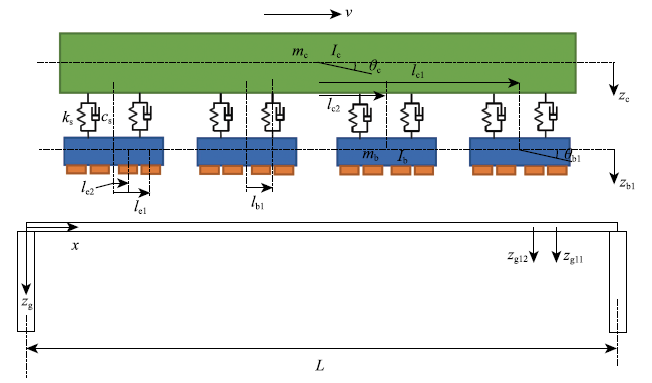Fig.1   Maglev vehicle/guideway coupling dynamic model

### 1.2 车辆振动方程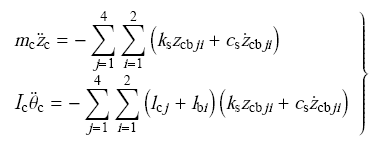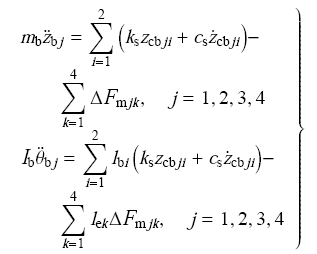$\left. \begin{array}{l} z_{{\rm cb}ji} = z_{\rm c} + \left( {l_{{\rm c}j} + l_{{\rm b}i} } \right)\theta _{\rm c}-z_{{\rm b}j}-l_{{\rm b}i} \theta _{{\rm b}j} \\ \dot {z}_{{\rm cb}ji} = \dot {z}_{\rm c} + \left( {l_{{\rm c}j} + l_{{\rm b}i} } \right)\dot {\theta }_{\rm c}-\dot {z}_{{\rm b}j}-l_{{\rm b}i} \dot {\theta }_{{\rm b}j} \\ \end{array}\right\}$

### 1.3 导轨振动方程

$m_{\rm g} \ddot {z}_{\rm g} + c_{\rm g} \dot {z}_{\rm g} + EI\frac{\partial ^4z_{\rm g} }{\partial x^4} = F\left( {x,t} \right)$

$\left. \begin{array}{l} z_{\rm g} \left( {0,t} \right) = z_{\rm g} \left( {L,t} \right) = 0 \\ EIz_{\rm g} ^{\prime \prime }\left( {0,t} \right) = EIz_{\rm g} ^{\prime \prime }\left( {L,t} \right) = 0 \\ \end{array}\right\}$

$$F\left( {x,t} \right) = \sum\limits_{j = 1}^4 \sum\limits_{k = 1}^4 \bigg\{ F_{{\rm m}jk} \cdot \delta \left( {x-x_{jk} } \right)\cdot\\ \left[ {H\left( {t-t_{jk} } \right)-H\left( {t-t_{jk}-\frac{L}{v}} \right)} \right] \bigg\}$$

$F_{{\rm m}jk} = F_{{\rm m}0} + \Delta F_{{\rm m}jk} ,F_{{\rm m}0} = \frac{m_{\rm c} g}{16} + \frac{m_{\rm b} g}{4}$

$\delta$($\cdot )$是Dirac函数

$\delta = \left\{ {\begin{array}{ll} 1,& x = x_{jk} \\ 0,& x \ne x_{jk} \\ \end{array}} \right.$

$H(t)$是单位阶跃函数

$H\left( t \right) = \left\{ {\begin{array}{lll} 1,& t > 0 \\ 0, & t < 0 \\ \end{array}} \right.$

$z_{\rm g} \left( {x,t} \right) = \sum\limits_{n = 1} {q_n \left( t \right)\sin \frac{nπ x}{L}}$

$\ddot {q}_n + 2\zeta _n \omega _n \dot {q}_n + \omega _n ^2q_n = p_n$

$$\zeta _n = \frac{c_{\rm g} }{2m_{\rm g} \omega _n } , \omega _n = \sqrt {\frac{EI}{m_{\rm g} }} \left( {\frac{nπ }{L}} \right)^2$$

$${\left. \begin{array}{l} p_n = \frac{2}{L}\sum\limits_{j = 1}^4 {\sum\limits_{k = 1}^4 {\left[ {F_{{\rm m}jk} \cdot \psi _n \left( {v,t} \right)} \right]} } \\ \psi _n \left( {v,t} \right) = \sin \left[ {\frac{nπ v\left( {t-t_{jk} } \right)}{L}} \right]\cdot \\ \qquad \left[ {H\left( {t-t_{jk} } \right)-H\left( {t-t_{jk}-\frac{L}{v}} \right)} \right] \\ \end{array}\right\}}$$

### 1.4 电磁力

$F_{{\rm m}jk} = \frac{\mu _0 A_{\rm m} N_{\rm m} ^2\left[ {I_0 + i_{jk} \left( t \right)} \right]^2}{4\left[ {\delta _0 + s_{jk} \left( t \right)} \right]^2}$

$\left. \begin{array}{l} F_{{\rm m}jk} = F_{{\rm m}0} + \Delta F_{{\rm m}jk} \\[1mm] F_{{\rm m}0} = \frac{m_{\rm c} g}{16} + \frac{m_{\rm b} g}{4} \\[1mm] \Delta F_{{\rm m}jk} = k_i \cdot i_{jk} \left( t \right)-k_{\rm c} \cdot s_{jk} \left( t \right) \\ s_{jk} \left( t \right) = z_{{\rm b}j} \left( t \right) + l_{{\rm e}k} \cdot \theta _{{\rm b}j} \left( t \right)-z_{{\rm g}jk} \left( t \right) \\ \end{array}\right\}$

$\left. \begin{array}{l} k_i = \frac{\mu _0 A_{\rm m} N_{\rm m} ^2I_0 }{2\delta _0 ^2} \\[3mm] k_{\rm c} = \frac{\mu _0 A_{\rm m} N_{\rm m} ^2I_0 ^2}{2\delta _0 ^3} \\[3mm] I_0 = \frac{2\delta _0 }{N_{\rm m} }\sqrt {\frac{F_{{\rm m}0} }{\mu _0 A_{\rm m} }} \\ \end{array}\right\}$

$z_{{\rm g}jk}$是电磁铁位置处导轨梁的扰度,用下式计算

$z_{{\rm g}jk} = \sum\limits_{n = 1} {q_n \left( t \right)\sin \frac{nπ x_{jk} }{L} = } \sum\limits_{n = 1} {q_n \left( t \right)\sin \frac{nπ v\left( {t-t_{jk} } \right)}{L}}$

### 1.5 车轨耦合系统方程及求解

$\left. \begin{array}{l} \dot {x}\left( t \right) = A\left( t \right)x\left( t \right) \\ x = \left\{ {\begin{array}{l} z_{\rm c} ,\dot {z}_{\rm c} ,\theta _{\rm c} ,\dot {\theta }_{\rm c} ,z_{\rm b1} ,\dot {z}_{\rm b1} ,\theta _{\rm b1} ,\dot {\theta }_{\rm b1} , \cdots , \\ z_{\rm b4} ,\dot {z}_{\rm b4} ,\theta _{\rm b4} ,\dot {\theta }_{\rm b4} ,q_1 , \cdots ,q_n ,\dot {q}_1 , \cdots ,\dot {q}_n \\ \end{array}} \right\}^{\rm T} \\ \end{array}\right\}$

## 2 时滞在计算过程中处理方法

$i_{jk} \left( t \right) = k_{\rm p} \cdot s_{jk} \left( {t-\tau _{\rm p} } \right) + k_{\rm d} \cdot \dot {z}_{jk}$

## 3 结果分析

### 3.1 设计参数

Table 1  Vehicle parameters

mc/kg,c/(kg.m2)mb/kgIb/(kg.m2)
29200140160080006500
ks/(N.m-1)Cs/(Ns.m-1)(lc1,-lc4)/m(lc2,-lc3)/m
8.515X1041.058X10493
lb1 /mlb2/m(le1,-le4)/m(le2,-le3)/m
1.5-1.52.250.75

Table 2  Guideway parameters

L/mNmodemg/(kg.m-1)E"(N.m-1)Zn
251237602.456X10100.05

### 图2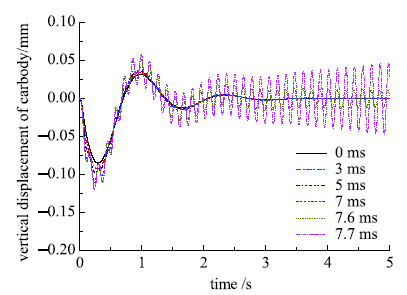Fig.2   Time history of carbody motion (rigid guideway)

### 图3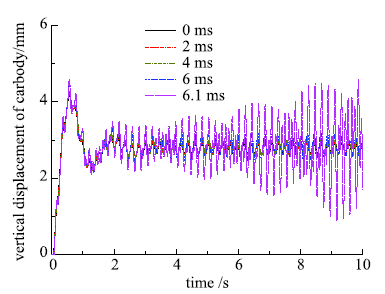Fig.3   Time history of carbody motion (flexible guideway)

### 图4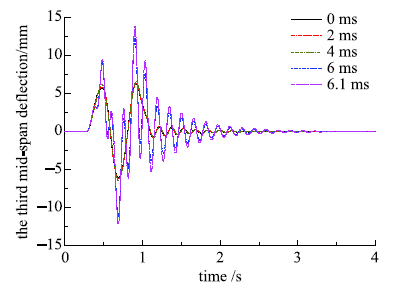Fig.4   Time history of the third mid-span deflection

### 图5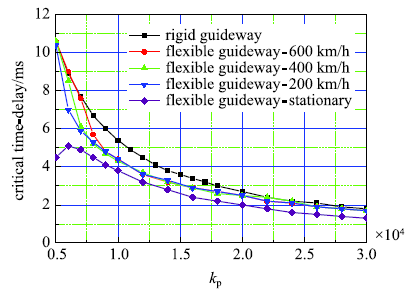Fig.5   Relationship between the critical time delay with clearance feedback control gain

### 图6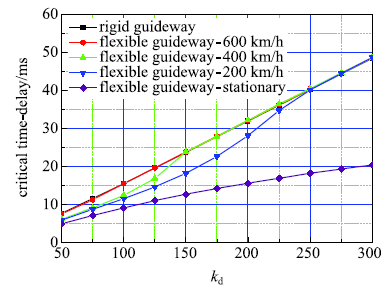Fig.6   Relationship between the critical time delay with velocity feedback control gain

### 图7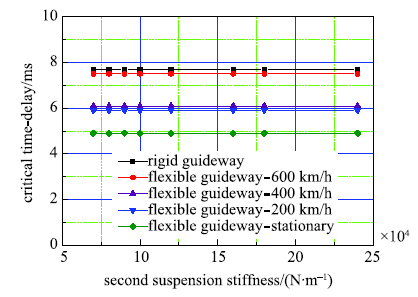Fig.7   Relationship between the critical time delay with second suspension stiffness

### 图8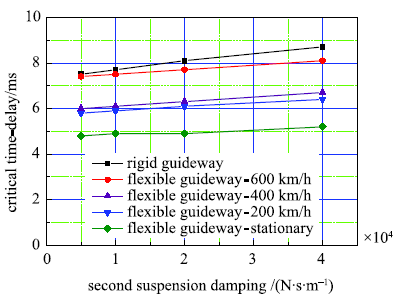Fig.8   Relationship between the critical time delay with second suspension damping

### 图9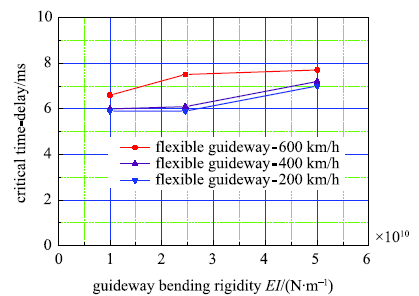Fig.9   Relationship between the critical time delay with guideway bending rigidity

### 图10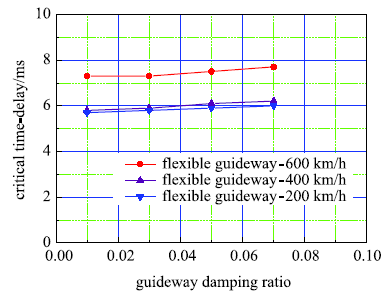Fig.10   Relationship between the critical time delay with guideway damping ratio

## 4 结 论

(1)增大导轨弯曲刚度和阻尼比;

(2)减小间隙反馈控制增益;

(3)增大速度反馈控制增益;

(4)增大二系悬挂阻尼.

The authors have declared that no competing interests exist.

## 参考文献 原文顺序 文献年度倒序 文中引用次数倒序 被引期刊影响因子

Lee HW, Kim KC, Lee J .

Review of maglev train technologies

IEEE Transactions on Magnetics, 2006,42(7):1917-1925

URL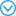This paper reviews and summarizes Maglev train technologies from an electrical engineering point of view and assimilates the results of works over the past three decades carried out all over the world. Many researches and developments concerning the Maglev train have been accomplished; however, they are not always easy to understand. The purpose of this paper is to make the Maglev train technologies clear at a glance. Included are general understandings, technologies, and worldwide practical projects. Further research needs are also addressed.

URL( Zhai Wanming, Zhao Chunfa .

Dynamics of maglev vehicle/guideway systems (I)-magnet/rail interaction and system stability

Chinese Journal of Mechanical Engineering, 2005,41(7):1-10 (in Chinese))

URLURL     Magsci( Shi Jin, Wei Qingchao, Wan Chuanfeng , et al.

Dynamic response of maglev vehicle track beam under random irregularity

Chinese Journal of Theoretical and Applied Mechanics, 2006,38(6):850-857 (in Chinese))

URL     MagsciZhao CF, Zhai WM .

Maglev vehicle guideway vertical random response and ride quality

Vehicle System Dynamics, 2002,38(3):185-210

URLSummary The research and development (R & D) of maglev technology had made a great progress in China since the early 1980s. Especially, a 35 km-long Shanghai high-speed maglev railway employing the German Transrapid system began to be constructed on March 1, 2001. Based on the Transrapid system, the paper develops a 10-degree-of-freedom model of maglev vehicle running over three types of guideways with constant speed. Random guideway irregularities are discussed and taken into account for simulation of the vehicle response and for evaluation of the ride comfort. Using the direct time integration method and the discrete fast Fourier transform (DFFT), random responses of the maglev vehicle-guideway systems are obtained and analyzed. Numerical results show that the resonant frequency of car body acceleration response is 0.5 1 Hz, and there is a 2.2 Hz periodic vibration due to the periodic configuration of rigid piers when the maglev vehicle travels over the elevated-beam guideway. The car body acceleration power spectral density (PSD) curves meet well the ride quality criterion of the urban tracked aircushion vehicle (UTACV) and the maximum acceleration of car body is less than 0.05 g. Moreover, the Sperling ride index values are less than 2.5 as long as the operational speed is less than 450 km/h. It is concluded that the maglev vehicle ride quality is quite well.

Wu H, Zeng XH, Yu Y .

Motion stability of high-speed maglev systems in consideration of aerodynamic effect: A study of a single magnetic suspension system

Acta Mechanica Sinica, 2017,33(6):1084-1094

URLIn this study, the intrinsic mechanism of aerodynamic effects on the motion stability of a high-speed maglev system was investigated. The concept of a critical speed for maglev vehicles considering the aerodynamic effect is proposed. The study was carried out based on a single magnetic suspension system, which is convenient for proposing relevant concepts and obtaining explicit expressions. This study shows that the motion stability of the suspension system is closely related to the vehicle speed when aerodynamic effects are considered. With increases of the vehicle speed, the stability behavior of the system changes. At a certain vehicle speed,the stability of the system reaches a critical state, followed by instability. The speed corresponding to the critical state is the critical speed. Analysis reveals that when the system reaches the critical state, it takes two forms, with two critical speeds, and thus two expressions for the critical speed are obtained. The conditions of the existence of the critical speed were determined, and the effects of the control parameters and the lift coefficient on the critical speed were analyzed by numerical analysis. The results show that the first critical speed appears when the aerodynamic force is upward,and the second critical speed appears when the aerodynamic force is downward. Moreover, both critical speeds decrease with the increase of the lift coefficient.

Han HS, Yim BH, Lee JK , et al.

Effects of guideway's vibration characteristics on the dynamics of a maglev vehicle

Vehicle System Dynamics, 2009,47(3):309-324

URLThe levitation control system in an electromagnetically levitated vehicle controls the voltage in its winding to maintain the air gap, which is the clearance between the electromagnet and the guideway, within an allowable range of variation, while strongly interacting with the flexible guideway. Thus, the vibrational characteristics of the guideway play an important role in the dynamics of Maglev (magnetically levitated) vehicles that utilise an active electromagnetic suspension system. In this study, the effects of the guideway''s vibrational characteristics, such as natural frequency and damping, on the dynamics of the Maglev vehicle UTM-02 are numerically and experimentally analysed. From these analyses, the coupled equations of motion of the simplified vehicle guideway model with three degrees of freedom are derived. Eigenvalues are calculated and frequency response analysis is also performed, in order to obtain a clear understanding of the dynamic characteristics resulting from the guideway''s vibrational characteristics. To verify the numerical results, air gap tests of the urban Maglev vehicle UTM-02 are also carried out. These results lead us to recommend that the natural frequency of the guideway be decreased by increasing mass density rather than by decreasing rigidity, and that its damping ratio be increased in the Maglev vehicle UTM-02 employing a five-state feedback control law as a levitation control law.

Han HS, Yim BH, Lee NJ , et al.

Prediction of ride quality of a Maglev vehicle using a full vehicle multi-body dynamic model

Vehicle System Dynamics, 2009,47(10):1271-1286

URLIn magnetically levitated (Maglev) transportation systems, especially in electromagnetic suspension system (EMS) type Maglev systems, highly accurate prediction of ride quality is very important in order to reasonably relax guideway construction tolerances or constraints and stiffness while meeting the specification for ride comfort, thereby reducing guideway construction and maintenance costs. A full vehicle multi-body dynamic model is proposed, to facilitate a rigorous ride quality prediction of an EMS-type Maglev vehicle. Using the more realistic dynamic model proposed in this paper, the effects of guideway deflection limits, surface roughness, and levitation control system parameters on ride quality are studied numerically. The results obtained from the simulation studies are then used to facilitate a discussion of the trade-off between guideway smoothness and vehicle suspension. It can be expected that these studies could suggest cost-effective specifications for guideway construction tolerances and stiffness and EMS.

Yim BH, Han HS, Lee JK , et al.

Curving performance simulation of an EMS-type maglev vehicle

Vehicle System Dynamics, 2009,47(10):1287-1304

URLFor electromagnetic suspension (EMS) type urban Maglev vehicles using a U-shaped electromagnet, the levitation and guidance forces are generated by only one electromagnet. Although the levitation force is actively controlled by changing the voltage of the electromagnet, the guidance force is passively determined by the levitation force. In addition, the curve negotiation performance of EMS-type urban Maglev vehicles using a U-shaped electromagnet must be considered, because an urban guideway may have some curves with shorter radii. It is, therefore, necessary to predict the curving performance with the greatest accuracy possible, in order to improve electromagnetic suspension and establish guideway design specifications. The objective is to establish a new dynamic modelling technique, so as to achieve more realistic curving simulation and thus to more accurately evaluate the curving performance of an EMS-type Maglev vehicle. The use of a full vehicle multibody dynamic model is proposed, and is applied to the evaluation of curving performance. Design changes are also investigated to obtain the bogie design directions for minimising variation in the lateral air gap, which is a criterion for curving performance.

Lee JS, Kwon SD, Kim MY , et al.

A parametric study on the dynamics of urban transit maglev vehicle running on flexible guideway bridges

Journal of Sound and Vibration, 2009,328(3):301-317

URLThe purpose of this study is to develop a numerical model for a dynamic interaction analysis of an actively controlled maglev vehicle and flexible guideway structure. In addition, an investigation is performed of the effect of vehicle and guideway characteristics on dynamic responses of low and medium speed maglev systems. Dynamic governing equations are derived by combining the 5-dof maglev vehicle model, the modal properties of guideway structures and a LQG controller for electromagnetic suspension. An investigation is then carried out on the effect of vehicle models, vehicle speed, irregularity, guideway deflection ratio, span length, span continuity and damping ratio on dynamic responses of the relatively low speed maglev vehicle and guideway structures. From the numerical simulation, it is found that the air gap of the vehicle is strongly affected by vehicle speed, tract roughness and guideway deflection ratio. In particular, the guideway deflection ratio is the most influential parameter which governs the air gap. Continuous span girders are found to be effective in reducing the air gap because of its smooth curvature and small deflection slope near the support. However, the span length and damping ratio of the guideway structure do not affect the air gap. The overall dynamic magnification factor of the guideway girder is not severe compared to the traditional wheel type vehicle.

Kim KJ, Han JB, Han HS , et al.

Coupled vibration analysis of Maglev vehicle-guideway while standing still or moving at low speeds

Vehicle System Dynamics, 2015,53(4):587-601Dynamic instability, that is, resonance, may occur on an electromagnetic suspension-type Maglev that runs over the elevated guideway, particularly at very low speeds, due to the flexibility of the guideway. An analysis of the dynamic interaction between the vehicle and guideway is required at the design stage to investigate such instability, setting slender guideway in design direction for reducing construction costs. In addition, it is essential to design an effective control algorithm to solve the problem of instability. In this article, a more detailed model for the dynamic interaction of vehicle/guideway is proposed. The proposed model incorporates a 3D full vehicle model based on virtual prototyping, flexible guideway by a modal superposition method and levitation electromagnets including feedback controller into an integrated model. By applying the proposed model to an urban Maglev vehicle newly developed for commercial application, an analysis of the instability phenomenon and an investigation of air gap control performance are carried out through a simulation.

Zhou DF, Hansen CH, Li J .

Suppression of maglev vehicle-girder self-excited vibration using a virtual tuned mass damper

Journal of Sound and Vibration, 2011,330(5):883-901

URLThe self-excited vibration that occurs between a stationary Electromagnetic Suspension (EMS) maglev vehicle and a girder is a practical problem that greatly degrades the performance of a maglev system. As of today, this problem has not been fully solved. In this article, the principle underlying the self-excited vibration problem is explored, and it is found that the fundamental resonance frequency of the maglev girder plays a vital role in the initiation of the self-excited vibration. To suppress the self-excited vibration, a scheme applying a tuned mass damper (TMD) to the maglev girder is proposed, and the stability of the combined system is analyzed. Furthermore, a novel concept of a virtual TMD is introduced, which uses an electromagnetic force to emulate the force of a real TMD acting on the girder. However, in the presence of the time delay caused by the inductance of the electromagnets, the stability analysis of the levitation system combined with the virtual TMD becomes complex. Analysis of the stability shows that there exist some repeated time delay zones within which the overall system is stable. Based on this rule, time delay control is introduced to stabilize the system with a virtual TMD, and a procedure to determine the optimal time delay and gain is proposed. Numerical simulation indicates that the proposed virtual TMD scheme can significantly suppress the self-excited vibration caused by one unstable vibration mode, and is suitable for application to EMS maglev systems.

Zhou DF, Li J, Hansen CH .

Application of least mean square algorithm to suppression of maglev track-induced self-excited vibration

Journal of Sound and Vibration, 2011,330(24):5791-5811

URL78 We construct the maglev electromagnet–track coupled model and examine its stability. 78 We employ the harmonic balance method to investigate the amplitude of the vibration. 78 An adaptive LMS algorithm with phase correction is employed to cancel the vibration. 78 The analysis shows that the presented algorithm can totally eliminate the vibration. 78 The presented algorithm is validated by numerical simulation and experiments.

Zhou DF, Li J, Zhang K .

Amplitude control of the track-induced self-excited vibration for a maglev system

ISA Transactions, 2014,53(5):1463-1469

URL61This paper investigates the principle of the track-induced self-excited vibration.61The amplitude of the vibration is investigated using the harmonic balance method.61We discover that the amplitude of the vibration is mainly decided by the voltage of the power supplier.61We provide a simple scheme to suppress the track-induced self-excited vibration.

Li JH, Li J, Zhou DF , et al.

The active control of maglev stationary self-excited vibration with a virtual energy harvester

IEEE Transactions on Industrial Electronics, 2015,62(5):2942-2951

URLThis paper addresses the active control of stationary self-excited vibration, which degrades the stability of the levitation control, decreases the ride comfort, and restricts the construction cost of the maglev system. First, a minimum interaction model containing a flexible bridge and a single levitation unit is presented. Based on the minimum interaction model, the principle underlying the self-excited vibration is explored. It shows that the active property of the levitation system is the root of self-excited vibration. Consider that the energy of vibration may be absorbed by the electromagnetic energy harvester (EEH), so that a technique applying it to the bridge is proposed, and the stability of the combined system is analyzed. However, its hardware structure is complicated, and the cost of construction is prohibitive. Then the novel conception of the virtual EEH is brought forward, which uses the electromagnetic force to emulate the force of a real energy harvester acting on the bridge. With the estimation of the vertical velocity of the bridge and the frequency of vibration, the self-oscillatory is avoided as well by adding an extra control instruction to the electromagnet. After building the overall dynamic model with details, numerical simulations and field experiments are carried out, and the results illustrating the improvement of stability are provided and analyzed.

( Wang Hongpo . Vehicle-Guideway Dynamic Interaction of the EMS Low Speed Maglev Vehicle. [PhD Thesis]. Changsha: National University of Defense Technology, 2007 (in Chinese))

Xu J Q, Chen C, Gao D G , et al.

Nonlinear dynamic analysis on maglev train system with flexible guideway and double time-delay feedback control

Journal of Vibroengineering, 2017,19(8):6346-6362

URL( Zhang Shu, Xu Jian .

Review on nonlinear dynamics in system with coupling delays

Chinese Journal of Theoretical Applied Mechnaics, 2017,49(3):565-587 (in Chinese))

URLURL( Li Shuai, Zhou Jilei, Ren Chuanbo , et al.

The research of time delay vibration control with time-varying parameters

Chinese Journal of Theoretical and Applied Mechanics, 2018,50(1):99-108 (in Chinese))

URLURL( Gong Xulu, Xu Pengfei .

Stochastic resonance of a memorial-damped system with time delay feedback and fluctuating mass

Chinese Journal of Theoretical and Applied Mechanics, 2018,50(4):880-889 (in Chinese))

URLLi JH, Li J, Zhou DF , et al.

Self-excited vibration problems of maglev vehicle-bridge interaction system

Journal of Central South University, 2014,21(11):4184-4192

URLThe self-excited vibration problems of maglev vehicle-bridge interaction system were addressed, which greatly degrades the stability of the levitation control, decreases the ride comfort, and restricts the cost of the whole system. Firstly, the coupled model containing the quintessential parts was built, and the mechanism of self-excited vibration was explained in terms of energy transmission from levitation system to bridge. Then, the influences of the parameters of the widely used integral-type proportion and derivation(PD) controller and the delay of signals on the stability of the interaction system were analyzed. The result shows that the integral-type PD control is a nonoptimal approach to solve the self-excited vibration completely. Furthermore, the differential-type PD controller can guarantee the passivity of levitation system at full band. However, the differentiation of levitation gap should be filtered by a low-pass filter due to noise of gap differentiation. The analysis indicates that a well tuned low-pass filter can still keep the coupled system stable.

Wang HP, Li J, Zhang K .

Stability and Hopf bifurcation of the maglev system with delayed speed feedback control

Acta Automatica Sinica, 2007,33(8):829-834

URLThe problem of time delay speed feedback in the control loop is considered here. Its effects on the linear stability and dynamic behavior of the maglev system are investigated. It is found that a Hopf bifurcation can take place when the time delay exceeds certain values. The stability condition of the maglev system with the time delay is acquired. The direction and stability of the Hopf bifurcation are determined by constructing a center manifold and by applying the normal form method. Finally, numerical simulations are performed to verify the analytical result.

Wang HP, Li J, Zhang K .

Non-resonant response, bifurcation and oscillation suppression of a non-autonomous system with delayed position feedback control

Nonlinear Dynamics, 2008,51(3):447-464

URLThe maglev system with delayed position feedback control is excitated by the deflection of flexible guideway and resonant response may take place. This paper concerns the non-resonant response of the system by employing centre manifold reduction and method of multiple time scales. The dynamical model is presented and expanded to the third-order Taylor series. Taking time delay as its bifurcation parameter, the condition with which the Hopf bifurcation may occur is investigated. Centre manifold reduction is applied to get the Poincar茅 normal form of the nonlinear system so that we can study the relationship between periodic solution and system parameter. At first, the non-resonant periodic solution of the normal form is calculated based on the method of multiple time scales. Then the bifurcation condition of the free oscillation in the solution is analyzed, and we get the conditions with which the free oscillation has maximum and minimum values. The relationship between external excitation and the periodic solution is also discussed in this paper. Finally, numerical simulation results show how system and excitation parameters affect the system response. It is shown that the existence of the free oscillation and the amplitude of the forced oscillation can be determined by time delay and control parameters. So felicitously selecting them can suppress the oscillation effectively.

Wang HP, Li J, Zhang K .

Sup-resonant response of a non-autonomous maglev system with delayed acceleration feedback control

IEEE Transactions on Magnetics, 2008,44(10):2338-2350

URLA Maglev system with delayed acceleration feedback control is disturbed by the deflection of flexible guideway, and resonant response may take place. We have investigated sup-resonant response of the Maglev system by employing center manifold reduction and the method of multiple scales. We present the dynamic model and expand it to a third-order Taylor series. Taking time delay as its bifurcation parameter, we discuss the condition for the occurring of Hopf bifurcation. We apply center manifold reduction to get the Poincare normal form of the nonlinear system and employ the perturbation technique to study sup-resonant response of the system. This yields the sup-resonant periodic solution of the normal form. We analyze the stability condition of the free oscillation in the solution and discuss the relationship between guideway excitation and periodic solution. Finally, numerical results show how time delay, control, and excitation parameters affect the system response. With the proper system parameter, the free oscillation may vanish and only the periodic solution plays a part. Time delay can control amplitude of the forced oscillation. The appearance of the chaos phenomenon can also be governed by regulating time delay. And judiciously selecting a control parameter makes it possible to suppress the response.

Zhang LL, Campbell SA, Huang LH .

Nonlinear analysis of a maglev system with time-delayed feedback control

Physica D-Nonlinear Phenomena, 2011,240(21):1761-1770

URL78 We study a nonlinear model for a maglev system with time-delayed feedback control. 78 We give constraints on the feedback gains and delay to ensure that the control stabilizes the equilibrium. 78 We show that a Hopf bifurcation occurs when the equilibrium loses stability. 78 Using the method of multiple scales, we show that the Hopf bifurcation may be subcritical or supercritical. 78 We show that nonlinear instability exists—the equilibrium may only be stable to small perturbations.

Zhang LL, Huang LH, Zhang ZZ .

Stability and Hopf bifurcation of the maglev system with delayed position and speed feedback control

Nonlinear Dynamics, 2009,57(1-2):197-207

URLIn this paper, the dynamic behavior of suspension system of maglev train with time-delayed position and velocity feedback signal is considered with rigid guideway. The stability conditions of the system are obtained with characteristic root method. The Hopf bifurcation direction and stability of the system at the critical point are also investigated. Based on center manifold reduction and Poincar normal form theory, the general formula for the direction, the estimation formula of period and stability of Hopf bifurcating periodic solution are also given. It is shown that time delays can change the current complicated dynamic behavior. And the condition that the bifurcation may occur is given to restrain the dynamic response and vibration between vehicle and guideway of the system with time-delayed position and velocity signal.

Zhang LL, Zhang ZZ, Huang LH .

Double Hopf bifurcation of time-delayed feedback control for maglev system

Nonlinear Dynamics, 2012,69(3):961-967

URLAbstract This paper undertakes an analysis of a double Hopf bifurcation of a maglev system with time-delayed feedback. At the intersection point of the Hopf bifurcation curves in velocity feedback control gain and time delay space, the maglev system has a codimension 2 double Hopf bifurcation. To gain insight into the periodic solution which arises from the double Hopf bifurcation and the unfolding, we calculate the normal form of double Hopf bifurcation using the method of multiple scales. Numerical simulations are carried out with two pairs of feedback control parameters, which show different unfoldings of the maglev system and we verify the theoretical analysis.

Zhang ZZ, Zhang LL .

Hopf bifurcation of time-delayed feedback control for maglev system with flexible guideway

Applied Mathematics & Computation, 2013,219(11):6106-6112

URLThis paper considers flexible guideway system for maglev train with time-delayed velocity feedback control gain. Taking the time delay as a bifurcation parameter, the parameter condition that Hopf bifurcation occurs is deduced. The stability and direction of the bifurcation periodic solution are analyzed by applying the normal form theory and the center manifold theorem. Numerical simulation and experimental result demonstrate the complex behavior of maglev system and support the theoretical analysis.

Zhang LL, Huang JH, Huang LH , et al.

Stability and bifurcation analysis in a maglev system with multiple delays

International Journal of Bifurcation & Chaos, 2015, 25(5):1550074-1-1550074-9

URLThis paper considers the time-delayed feedback control for Maglev system with two discrete time delays. We determine constraints on the feedback time delays which ensure the stability of the Maglev system. An algorithm is developed for drawing a two-parametric bifurcation diagram with respect to two delays u03c41 and u03c42. Direction and stability of periodic solutions are also determined using the normal form method and center manifold theory by Hassard. The complex dynamical behavior of the Maglev system near the domain of stability is confirmed by exhaustive numerical simulation.

Zhang LL, Huang LH, Zhang ZZ .

Hopf bifurcation of the maglev time-delay feedback system via pseudo-oscillator analysis

Mathematical and Computer Modelling, 2010,52(5):667-673

URLThis paper investigates the local dynamics around the trivial solution of suspension system of maglev train with time-delayed feedback signals. With characteristic root method, the linear stability analysis of the maglev system is obtained, which implies that a Hopf bifurcation may occur when time delay exceeds a critical value. To gain insight into the periodic motion, the pseudo-oscillator analysis is used to calculate the bifurcated periodic solution, and to determine the direction of the bifurcation. Unlike the widely used methods such as manifold reduction, the pseudo-oscillator analysis involves simple computation and gives prediction of the local dynamics with high accuracy. Numerical simulation results show that the existence of the Hopf bifurcation and the amplitude of the periodic solution can be determined by time delay and control parameters. So appropriately selecting them can restrain vibration between vehicle and guideway of the system effectively.

/

 〈〉